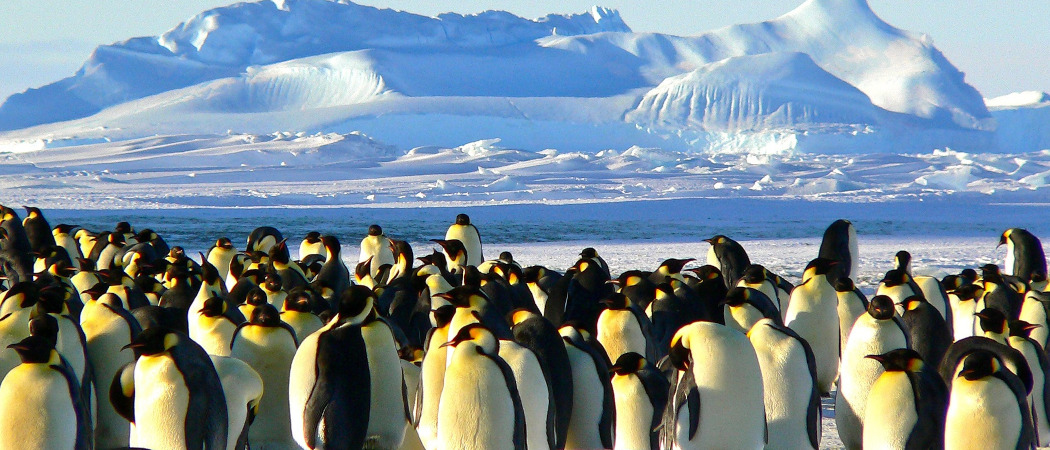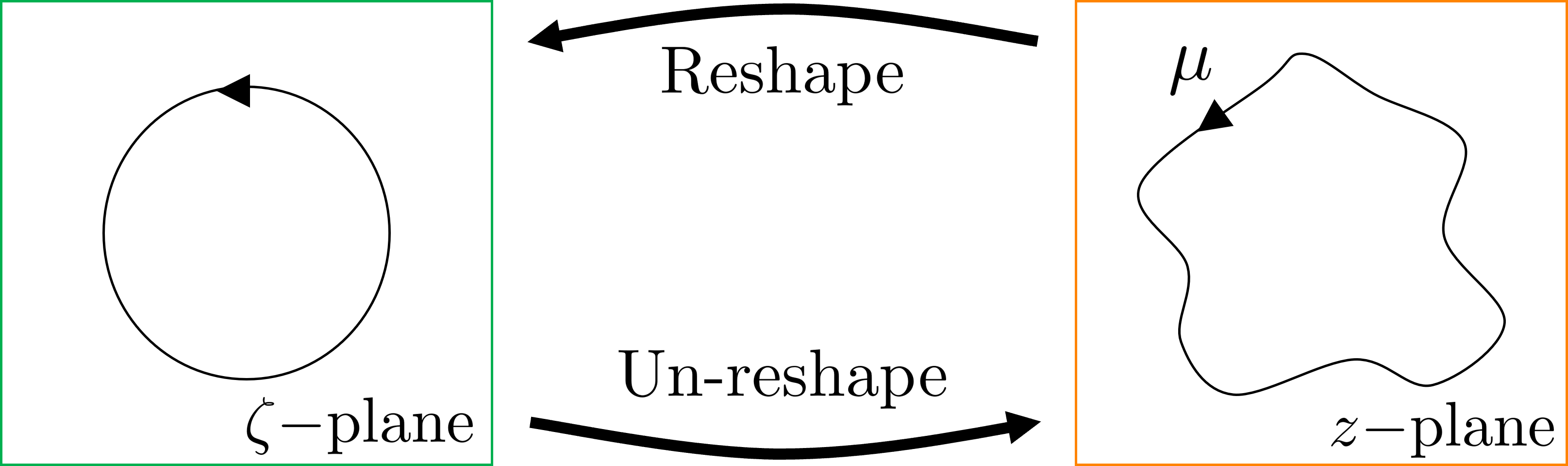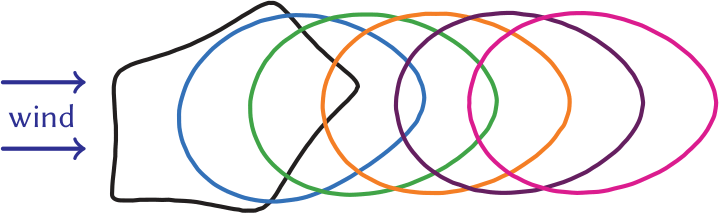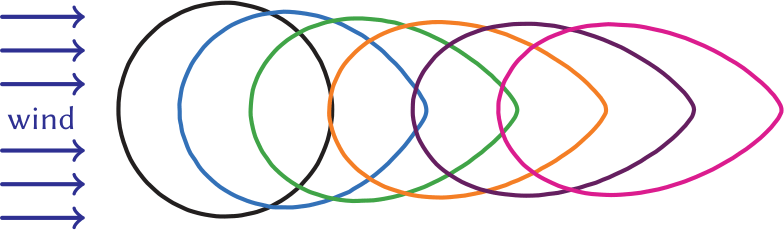# Penguins: the emperors of fluid dynamics

Sam Harris looks at how Pingu and his friends can stay cosyLet me guess, you like penguins? I mean, who doesn’t?! And I am guessing you like maths too? Well, what if I told you I could combine the two? Have I captured your interest?

Antarctic penguins experience some of the most extreme weather conditions on Earth, where wind speeds exceed 70mph and temperatures drop below -40°C. However, penguins have adopted an effective survival strategy. The whole colony comes together to form one large huddle to shield the birds from the wind and to conserve each penguin’s heat. While huddling, the birds are constantly waddling around, causing the entire huddle to move. But what are the underlying mechanisms driving this movement? And can we track the huddle’s movement over time?

## They’re hot, then they’re cold

To answer these questions, we need to view the problem more mathematically. Intuitively, we know that the penguins are huddling together for warmth, so let’s start by thinking in terms of heat and energy. In other words, let’s consider the thermodynamics of the problem. We identify two key heat transfer processes at work.

First, the penguins are losing heat to the cold air and the fast flow of the wind means that a temperature gradient—a difference in temperature between the air and the penguins—is always sustained. You will have experienced this yourself in everyday (non-Antarctic) life. On a windy day, you feel colder, right? This is because your body loses heat to the air, and the air you just warmed up moves on and is replaced by fresh, cold air for you to lose heat to again.

Second, the penguins are receiving heat from neighbouring birds in the huddle. Once again, think about your everyday life: imagine standing in a crowded queue waiting to buy the new Zelda game. Despite the cool company you are surrounded by, you feel hotter in the crowd as you’re gaining heat from the people around you. It is the same in a penguin huddle (except they can’t play the new Zelda game: their flippers can’t hold the controller). This heating is particularly effective near the huddle centre, where temperatures can reach up to 37.5°C: higher than the average human body temperature!

Unfortunately, this temperature is actually too high and can cause the penguins to overheat. To counteract this, the birds regularly reorganise themselves in the huddle: those in the centre move to the edge and vice versa. In other words, they are all massive Katy Perry fans—by reenacting the song Hot N Cold, all members of the huddle are kept at a nice, cosy average temperature.

We can start making a model of the huddle’s movement based on these two heat transfer processes. First though, let’s add one final condition: we assume that no penguins leave or join the huddle, so the size of the huddle, ie the number of penguins, is conserved. So, there are three things in total affecting the huddle’s movement: cooling by the wind, heating by the penguins and conservation of the huddle size. These three effects become important later, so keep them in mind!

## In love with the shape of $\mu$

Our aim is to see how the entire huddle moves over time. Therefore, instead of viewing the huddle as hundreds of individual penguins (a discrete model) we treat it as a continuous ‘blob’ of penguins (a continuum model).

Imagine looking down at the penguin huddle from above and tracing around its edge. The resulting curve is called the huddle boundary and we label it $\mu$. All the penguins are inside of $\mu$ so we call this the interior ‘penguin’ region $P$. The huddle is tight enough so that no wind passes through to $P$ and all wind flow is confined to the exterior ‘wind’ region $W$. Wind flowing over the top of the huddle just contributes a constant heat loss to every penguin; this will not affect how the huddle moves. Hence we can treat our problem as two-dimensional.Top down view of the huddleSide view of the huddle

This is an example of a free boundary problem which is exactly as it sounds: you want to find the motion of some boundary which is free to move wherever it chooses, ie its flow is not confined within walls or pipes. In this case, we want to find how the penguin huddle boundary $\mu$ moves, which would then tell us how the entire huddle moves.

We need to find the velocity $v$ of the curve $\mu$ in the outward normal direction—the direction marked by the unit normal vector $\hat{\boldsymbol{n}}$ in the ‘top down view’ diagram. Consider energy conservation: the (heat) energy lost by the penguins must be equal to that gained by the wind. From this idea, we get an equation for $v$, \begin{equation}v = \hat{\boldsymbol{n}}\cdot\boldsymbol{\nabla}T_W-\hat{\boldsymbol{n}}\cdot\boldsymbol{\nabla}T_P + A(t),\tag{⁂} \end{equation} where $\boldsymbol{\nabla}T$ is the temperature gradient in the wind/penguin regions $W/P$.

Looking at the right-hand side of this equation: the first term relates to the temperature of the wind, the second term relates to the temperature of the penguins and the third term, $A(t)$, ensures that the area enclosed by the curve $\mu$ is conserved. As if by magic, these three terms exactly represent the three huddle effects we defined earlier: cooling by the wind, heating by the penguins and conservation of huddle size, respectively!

In order to solve for $v$, we need to find the wind and penguin temperature gradients $\boldsymbol{\nabla}T_W$ and $\boldsymbol{\nabla}T_P$. The tricky part is that we need to solve three more equations in order to get these two terms. For $\boldsymbol{\nabla}T_W$, we must solve the wind flow and wind temperature equations and for $\boldsymbol{\nabla}T_P$, we need to solve the penguin temperature equation (given in the box below). Adding some suitable boundary conditions, we get the complete system of equations ready to be solved.

So, to get the normal velocity $v$ of the huddle boundary $\mu$, we have to solve three equations across two regions: the wind and penguin regions. The whole thing is starting to look a bit big and scary, so how can we deal with it?

## The circle of life

I love circles! You may think they are one-sided and pointless, but they have constant radius and infinite lines of symmetry: how can you not like that?! Penguins like circles too because there is a known solution to our huddle problem when the boundary $\mu$ is a circle. The issue is, penguins do not always huddle in circles, but any random boundary shape can always be smoothed out into a circle, right?

## Dealing with wind

The wind flow and wind temperature equations, respectively, are \begin{equation*} \nabla^2\phi=0,\;\;\;\;\;\;\;\; \textit{Pe}\,\boldsymbol{u}\cdot\boldsymbol{\nabla}T_W = \nabla^2 T_W, \end{equation*} where $\phi$ is defined such that its gradient $\boldsymbol{\nabla}\phi$ is the wind velocity $\boldsymbol{u}$, the Péclet number Pe is a measure of the ambient wind strength and $\nabla^2$ represents diffusion. The first equation defines the motion of the wind. The second defines the steady convection and diffusion of temperature in the wind.

The penguin temperature equation is \begin{equation*} \nabla^2T_P=-R, \end{equation*} which represents the diffusion of temperature in the penguin huddle, where $R$ is the rate at which each penguin generates heat.

Let’s take our huddle boundary. We reshape it into a circle and find the known solution. Then we just ‘un-reshape’ the curve back to the original geometry and in this ‘un-reshaping’ step, we also transform the known solution. This process is, in fact, a mathematical method known as conformal mapping which uses a theorem called the Riemann mapping theorem.

Let’s say that the transformation, or conformal map, from the circle to the penguin huddle boundary $\mu$ is given by $f(\zeta,t)$, where $\zeta$ represents the coordinate system for the circle and $t$ is time. Our whole system of equations can be combined into one single equation in terms of $f$,

\begin{equation}\frac{\operatorname{Re}\Big[f_t\overline{\zeta f_\zeta}\Big]}{|f_\zeta|} = C-H+A(t),\tag{‽} \end{equation}
where $f_\zeta$ and $f_t$ are partial derivatives of $f$ with respect to $\zeta$ and $t$, $\operatorname{Re}[🐧]$ is the real part of $🐧$ and $\overline{🐧}$ is the complex conjugate of $🐧$. The equation looks complicated but the important point is, the left hand side of (‽) is just the normal velocity $v$ expressed in terms of the transformation $f$.

## Riemann mapping theorem

Consider the penguin huddle in the ‘$z$-plane’. There is always a transformation, or ‘conformal map’, from the huddle boundary $\mu$ in the $z$-plane to the unit circle in some other ‘$\zeta$-plane’, and vice versa. The conformal map can also transform the entire region exterior to the huddle boundary (the wind region) to the region exterior to the circle, and vice versa. The interior regions—the penguin region and the circle interior—are not additionally transformed.Note: the wind flow and wind temperature equations are unchanged in the conformal mapping process—they are ‘conformally invariant’. This makes things easier when finding the solution to our penguin huddle problem.

The right hand side of (‽) represents the three huddle effects: $C$ is the wind cooling effect, $H$ is the penguin heating effect and $A(t)$ is the area conservation effect as we saw in (⁂). These three terms can now be found numerically, ie via computer approximation, which involves much more work than this simple sentence suggests, but the margin is too small to write it all down.

Instead of solving our big, scary system of equations, now all we need to do is find the transformation $f(\zeta,t)$ from the circle to the huddle boundary. Once again, we can find $f$ numerically using any suitable programming language. I will be using the best one: Matlab. Sorry Python lovers, please consult Chalkdust issue 16’s big argument for my justification.

## How do you like your eggs in the… huddle

Now it’s picture time! Let’s see how a penguin huddle moves in response to a wind flowing horizontally from left to right.

First, let’s take an initially circular penguin huddle. Here is how the huddle moves:The shape outlined in black on the far left is the starting shape of the huddle—in this case a circle. Then each subsequent plot going right is how the huddle shape is changing over equal time increments. We notice two things: the huddle moves downwind and the boundary changes shape until eventually it becomes egg-shaped.

Let’s try something more complicated. We will keep all the conditions the same but, this time, the starting shape of the huddle will be a wonky pentagon. Its evolution looks like this:Again, the huddle moves downwind and its shape turns into an egg, the eggsact same egg that the previous huddle evolved into. In fact, regardless of the initial starting shape of the huddle boundary, the penguin huddle will always evolve into the same egg shape. Eggstraordinary!

Now, what happens if we increase the wind speed? Let’s take an initially circular huddle again:The huddle still turns into an egg, but the egg is much narrower now, like a tear drop. Eggsciting!

And finally, what happens if the effect of penguin heating is raised? Well then the huddle evolves like this:Now the final huddle shape is a very circular egg. Eggsceptional!

So, all huddle boundary starting shapes move downwind and turn into an egg shape. A higher wind speed gives a more streamlined egg, whereas an increased penguin heating effect gives a more circular egg. This is what we eggspect to see: penguin huddles in the real world move downwind and have similar boundary shapes as the ones we’ve shown in our pictures. Intuitively, it also makes sense for there to be some optimal huddle shape for minimising heat loss. So all these conclusions seem sunny side up to me.

## A whole new world… of free boundary problems

To sum everything up, penguin huddles move and change shape during cold winds to minimise the heat loss each penguin experiences. The two key thermodynamic processes, cooling by the wind and heating by neighbouring penguins, result in a whole system of equations governing the huddle’s movement. But the method of conformal mapping simplifies everything to a single equation, which means a numerical simulation of the huddle’s movement can be made.

Similar methods can be used to solve other free boundary problems. You could model other natural phenomena, such as water waves, melting ice or dissolving rocks. Even wildfires, the polar opposite of penguins, are free boundary problems and can be simulated in a similar way to penguin huddles. You could also simulate other animal groupings, like bird flocks, fish schools or insect swarms. Free boundary problems exist throughout nature and multidisciplinary mathematical tools are needed to solve them.

One final note. Lots of rigorous mathematics was needed for us to understand penguin huddles. But penguins have been forming huddles for millions of years. How did they figure it out? Perhaps huddling is just a natural instinct for them. Yes, that must be it. Or maybe not. Maybe they’ve known all along. Thermodynamics, conformal mapping, Matlab’s superiority over Python, all of it! Was Madagascar right? Are penguins smarter than us? What else have they been hiding? Wait, who’s that behind me? … I’ve said too much. You didn’t see anything.Sam is a PhD student at University College London with an interest in fluid dynamics and applied complex analysis. He and his supervisor, Robb McDonald, study free boundary problems in nature such as wildfire spread and, you guessed it, penguins. Sam also likes everything and anything Nintendo.